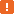# ValueByIndex Property### Description

The `ValueByIndex` property lets you obtain the value stored in the cell, which is specified by its column’s index.Since the test log layout is customized, the `ValueByIndex` property does not provide a reliable way to obtain the desired value. See Remarks for details.

### Declaration

LogTableRowObj.ValueByIndex(Index)

 LogTableRowObj An expression, variable or parameter that specifies a reference to a LogTableRow object Index [in] Required Integer

### Applies To

The property is applied to the following object:

### Parameters

The property has the following parameter:

Index

The index of the column to which the desired cell belongs. The index is zero-based, that is the first column has index 0, the second - 1, and so on. The index of the last column is `LogTableDataScheme.ColumnCount` - 1.

### Property Value

The value of the specified cell. It can be an integer or a floating-point number, a string, a boolean, a date-time value, a hyperlink or an image.

 Note: To determine the type of values in the given table column, you can use the `LogColumn.DataType` property.

### Remarks

If you use Python or DelphiScript, you should enclose the parameter of the `ValueByIndex` property in square brackets: `ValueByIndex[Index]`.It is not recommended to use the `ValueByIndex` property to obtain the value in the desired cell. This is because the index of the table column is dependent on the table layout, which is customized. So, the scripts which use the `ValueByIndex` property may not work correctly on another computer, or even on your computer if you change the log layout, for example, add or remove table columns or change their order. It is more reliable to use the `Value` or `ValueByName` property instead of `ValueByIndex`.

### Example

The following example demonstrates how to obtain the value stored in the specified cell.

JavaScript, JScript

function TableDataRowSample()
{
var Logs, LogItem, LogData, DType, Row, Column, Value;
// Obtains a log item
Logs = Project.Logs;
LogItem = Logs.LogItem(0);
// Obtains the log item’s dataset
LogData = LogItem.Data(0);

DType = LogData.Scheme.DataType;
// Checks whether the dataset corresponds to the table
if (DType == 0)
{
// Obtains a table row by its index
Row = LogData.Rows(0);
// Obtains the value stored in the cell by the column index
Value = Row.ValueByIndex(1);
// Posts the value to the test log
Log.Message(aqConvert.VarToStr(Value));
}

}

Python

``````def TableDataRowSample():
# Obtains a log item
Logs = Project.Logs
LogItem = Logs.LogItem
# Obtains the log item's dataset
LogData = LogItem.Data
DType = LogData.Scheme.DataType;
# Checks whether the dataset corresponds to the table
if DType == 0:
# Obtains a table row by its index
Row = LogData.Rows
# Obtains the value stored in the cell by the column index
Value = Row.ValueByIndex
# Posts the value to the test log
Log.Message(aqConvert.VarToStr(Value))``````

VBScript

Sub TableDataRowSample

' Obtains a log item
Set Logs = Project.Logs
Set LogItem = Logs.LogItem(0)
' Obtains the log item’s dataset
Set LogData = LogItem.Data(0)

DType = LogData.Scheme.DataType
' Checks whether the dataset corresponds to the table
If DType = 0 Then
' Obtains a table row by its index
Set Row = LogData.Rows(0)
' Obtains the value stored in the cell by the column index
Value = Row.ValueByIndex(1)
' Posts the value to the test log
Log.Message(aqConvert.VarToStr(Value))
End If

End Sub

DelphiScript

procedure TableDataRowSample();
var Logs, LogItem, LogData, DType, Row, Column, Value;
begin
// Obtains a log item
Logs := Project.Logs;
LogItem := Logs.LogItem;
// Obtains the log item’s dataset
LogData := LogItem.Data;

DType := LogData.Scheme.DataType;
// Checks whether the dataset corresponds to the table
if DType = 0 then
begin
// Obtains a table row by its index
Row := LogData.Rows;
// Obtains the value stored in the cell by the column index
Value := Row.ValueByIndex;
// Posts the value to the test log
Log.Message(aqConvert.VarToStr(Value));
end;

end;

C++Script, C#Script

function TableDataRowSample()
{
var Logs, LogItem, LogData, DType, Row, Column, Value;
// Obtains a log item
Logs = Project["Logs"];
LogItem = Logs.LogItem(0);
// Obtains the log item’s dataset
LogData = LogItem["Data"](0);

DType = LogData["Scheme"]["DataType"];
// Checks whether the dataset corresponds to the table
if (DType == 0)
{
// Obtains a table row by its index
Row = LogData["Rows"](0);
// Obtains the value stored in the cell by the column index
Value = Row["ValueByIndex"](1);
// Posts the value to the test log
Log["Message"](aqConvert["VarToStr"](Value));
}

}